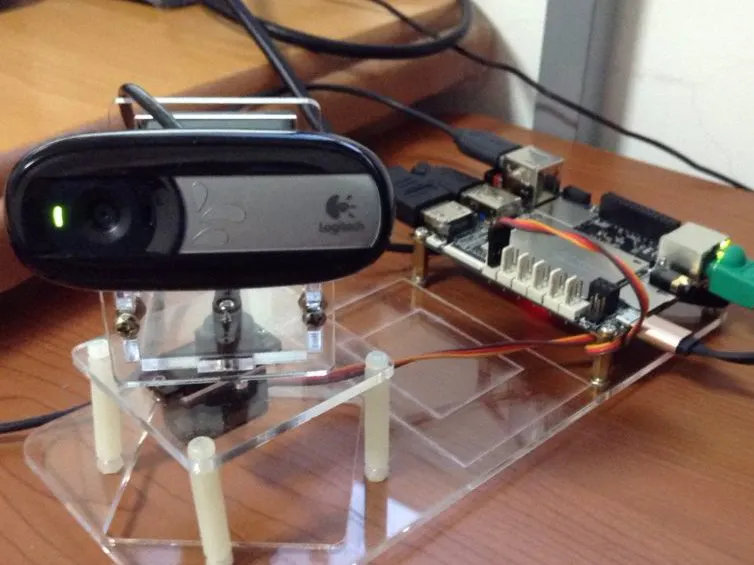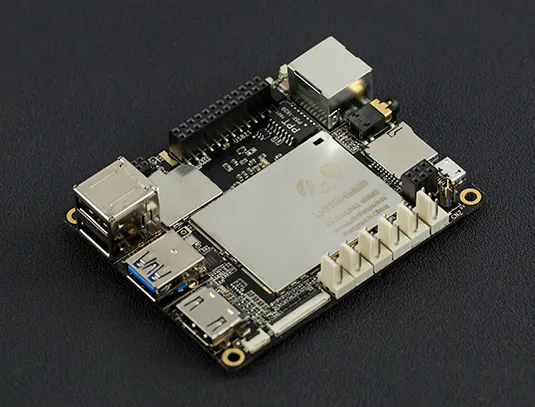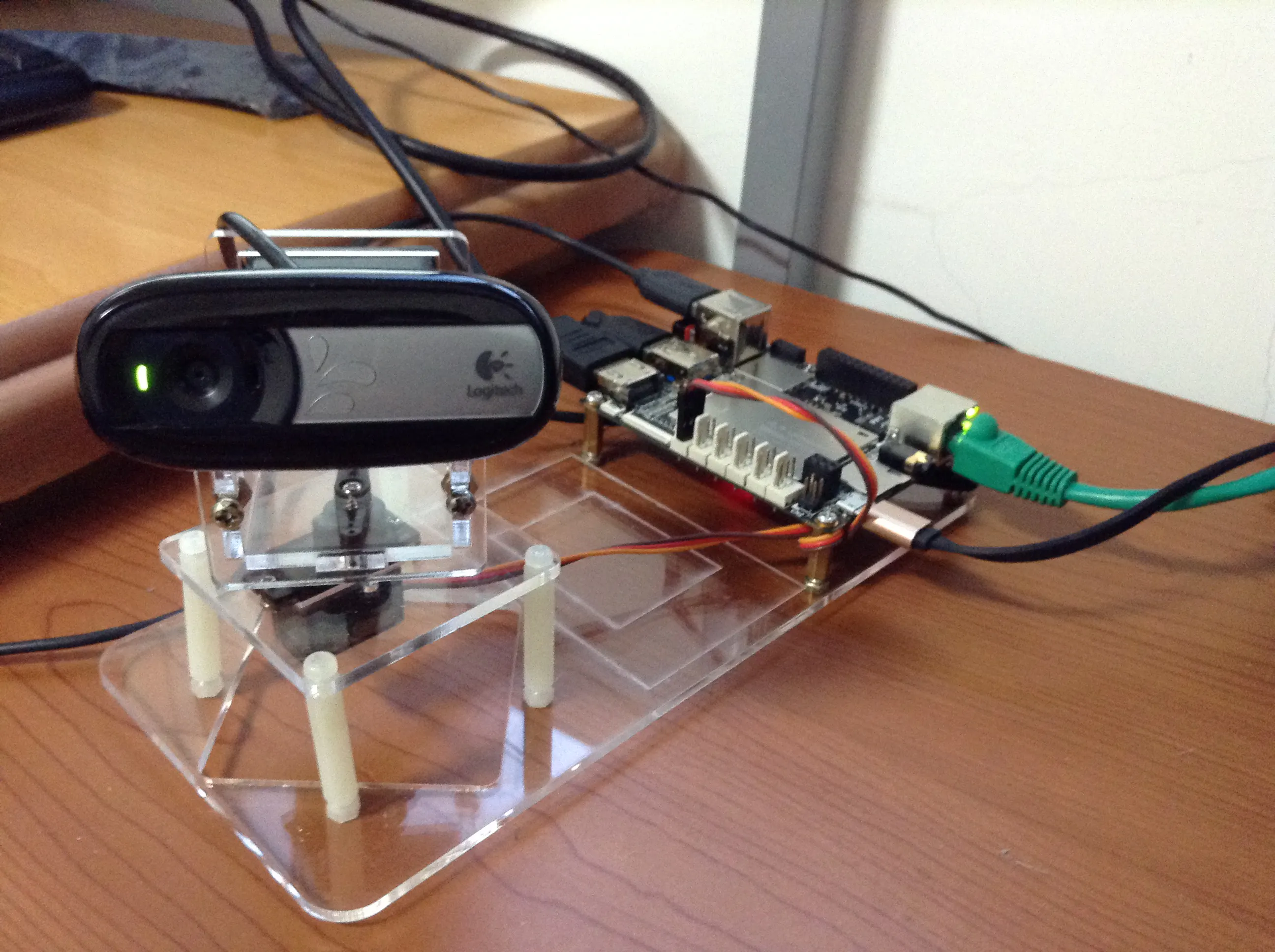# OpenCV Face Recognition with DFRobot LattePanda

This article shows how to use OpenCV visual processing library to build a face recognition robot with DFRobot LattePanda Windows SBC.## Things used in this project

### Hardware components

 Logitech C270 webcam or other USB webcam
×1LattePanda 4GB/64GB
×1
 MG90 servo (or other micro servo)
×1

### Software apps and online servicesOpenCV

## Schematics

### 1-1_WIPLi86Zaq.jpg

Physical setup of LattePanda, servo and webcam.## Code

### servo_control.ino

Arduino
Control servo angle by character sent from serial connection (Python)
```#include <Servo.h>

Servo s;
int degree = 90;

void setup()
{
Serial.begin(115200);
s.attach(9);
}

void loop()
{
if (Serial.available())
{
if (cmd == 'a')
{
degree++;
if (degree >= 180)
degree = 180;
s.write(degree);
}
else if (cmd == 'b')
{
degree--;
if (degree <= 0)
degree = 0;
s.write(degree);
}
else
Serial.println("Press a/b to turn the camrea!");
}
}
```

### openCV face recognition and send command to Arduino

Python
```import cv2
import numpy as np
import serial

cap = cv2.VideoCapture(0)
cap.set(3, 640)
cap.set(4, 480)
ser = serial.Serial('COM5', 115200)

while True:
gray = cv2.cvtColor(img, cv2.COLOR_BGR2GRAY)
if len(faces):
(x, y, w, h) = max(faces, key=lambda face: face*face)
cv2.rectangle(img,(x,y),(x+w,y+h),(255,0,0),2)
roi_gray = gray[y:y+h, x:x+w]
roi_color = img[y:y+h, x:x+w]
for (ex,ey,ew,eh) in eyes:
cv2.rectangle(roi_color,(ex,ey),(ex+ew,ey+eh),(0,255,0),2)
position = x + w/2.0
print(position)
if position < 320:
ser.write(b'a')
else:
ser.write(b'b')

cv2.imshow('face', img)
k = cv2.waitKey(5) & 0xFF
if k == 27:
break
cv2.destroyAllWindows()
```

## Credits

### CAVEDU Education

11 projects • 26 followers
We provide tutorials of robotics, Arduino, Raspberry Pi and IoT topics. http://www.cavedu.com

### DFRobot

57 projects • 127 followers
Empowering Creation for Future Innovators

### DFRobot Robotics

7 projects • 13 followers